Swapna S.

Btm Layout 2nd Stage, Bangalore, India - 560076Swapna S.

Btm Layout 2nd Stage, Bangalore, India - 560076.

3.9

UrbanPro Rating

Details verified of Swapna S.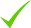IdentityEducation

Know how UrbanPro verifies Tutor details

Identity is verified based on matching the details uploaded by the Tutor with government databases.

Overview

Swapna S. conducts classes in Class 11 Tuition and Class 12 Tuition. Swapna is located in Btm Layout 2nd Stage, Bangalore. Swapna takes at students Home and Regular Classes- at her Home. She is well versed in English and Hindi.

Languages Spoken

English

Hindi

Btm Layout 2nd Stage, Bangalore, India - 560076

Verified Info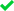Education VerifiedPhone Verified

Demo Class

No

Report this Profile

Is this listing inaccurate or duplicate? Any other problem?

Please tell us about the problem and we will fix it.

Please describe the problem that you see in this page.

Type the letters as shown below *

Please enter the letters as show below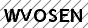Class 11 Tuition Overview

Class 11 Tuition

Class LocationStudent's HomeTutor's Home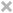Online (video chat via skype, google hangout etc)

Board

CBSE

CBSE Subjects taught

Accountancy

Taught in School or College

No

Reviews

this is test message this is test message this is test message this is test message this is test message this is test message this is test message

No Reviews yet! Be the first one to Review

FAQs

1. Which school boards of of Class 11 do you teach for?

CBSE

2. Have you ever taught in any School or College?

No

3. Which classes do you teach?

I teach Class 11 Tuition and Class 12 Tuition Classes.

4. Do you provide a demo class?

No, I don't provide a demo class.

5. How many years of experience do you have?

I have been teaching for less than a year.

Answers by Swapna S. (1068)

Answered 5 hrs ago CBSE/Class 12/Science/Physics/Unit 10-Communication Systems/COMMUNICATION SYSTEMS/NCERT Solutions/Exercise 15

For an amplitude modulated wave, the maximum amplitude is found to be 10 V while the minimum amplitude... ...more

For an amplitude modulated wave, the maximum amplitude is found to be 10 V while the minimum amplitude is found to be 2 V. Determine the modulation index μ. What would be the value of μ if the minimum amplitude is zero volt?

Maximum amplitude, Amax = 10 V Minimum amplitude, Amin = 2 V Modulation index μ, is given by the relation: ...more

Maximum amplitude, Amax = 10 V

Minimum amplitude, Amin = 2 V

Modulation index μ, is given by the relation:Dislike Bookmark

Answered 5 hrs ago CBSE/Class 12/Science/Physics/Unit 10-Communication Systems/COMMUNICATION SYSTEMS/NCERT Solutions/Exercise 15

A modulating signal is a square wave, as shown in Fig. 15.14. The carrier wave is given by (i)... ...more

A modulating signal is a square wave, as shown in Fig. 15.14.The carrier wave is given by(i) Sketch the amplitude modulated waveform

(ii) What is the modulation index?

It can be observed from the given modulating signal that the amplitude of the modulating signal, Am = 1 V It is given that the carrier wave c (t) = 2 sin (8πt) ∴Amplitude of the carrier wave, Ac = 2 V Time period of the modulating signal Tm = 1 s The angular frequency of the modulating signal... ...more

It can be observed from the given modulating signal that the amplitude of the modulating signal, Am = 1 V

It is given that the carrier wave c (t) = 2 sin (8πt)

∴Amplitude of the carrier wave, Ac = 2 V

Time period of the modulating signal Tm = 1 s

The angular frequency of the modulating signal is calculated as:The angular frequency of the carrier signal is calculated as:From equations (i) and (ii), we get:The amplitude modulated waveform of the modulating signal is shown in the following figure.(ii)Modulation index,Dislike Bookmark

Answered 5 hrs ago CBSE/Class 12/Science/Physics/Unit 10-Communication Systems/COMMUNICATION SYSTEMS/NCERT Solutions/Exercise 15

A carrier wave of peak voltage 12 V is used to transmit a message signal. What should be the peak... ...more

A carrier wave of peak voltage 12 V is used to transmit a message signal. What should be the peak voltage of the modulating signal in order to have a modulation index of 75%?

Amplitude of the carrier wave, Ac = 12 V Modulation index, m = 75% = 0.75 Amplitude of the modulating wave = Am Using the relation for modulation index: ...more

Amplitude of the carrier wave, Ac = 12 V

Modulation index, m = 75% = 0.75

Amplitude of the modulating wave = Am

Using the relation for modulation index:Dislike Bookmark

Answered 5 hrs ago CBSE/Class 12/Science/Physics/Unit 10-Communication Systems/COMMUNICATION SYSTEMS/NCERT Solutions/Exercise 15

Is it necessary for a transmitting antenna to be at the same height as that of the receiving antenna... ...more

Is it necessary for a transmitting antenna to be at the same height as that of the receiving antenna for line-of-sight communication? A TV transmitting antenna is 81m tall. How much service area can it cover if the receiving antenna is at the ground level?

Line-of-sight communication means that there is no physical obstruction between the transmitter and the receiver. In such communications it is not necessary for the transmitting and receiving antennas to be at the same height. Height of the given antenna, h = 81 m Radius of earth, R = 6.4 × 106... ...more

Line-of-sight communication means that there is no physical obstruction between the transmitter and the receiver. In such communications it is not necessary for the transmitting and receiving antennas to be at the same height.

Height of the given antenna, h = 81 m

Radius of earth, R = 6.4 × 106 m

For range, d = (2Rh)½, the service area of the antenna is given by the relation:

A = πd2

= π (2Rh)

= 3.14 × 2 × 6.4 × 106× 81

= 3255.55 × 106 m2

= 3255.55

∼ 3256 km2

Dislike Bookmark

Answered 5 hrs ago CBSE/Class 12/Science/Physics/Unit 10-Communication Systems/COMMUNICATION SYSTEMS/NCERT Solutions/Exercise 15

Digital signals (i) Do not provide a continuous set of values, (ii) Represent values as discrete... ...more

Digital signals

(i) Do not provide a continuous set of values,

(ii) Represent values as discrete steps,

(iii) Can utilize binary system, and

(iv) Can utilize decimal as well as binary systems.

Which of the above statements are true?

(a) (i) and (ii) only

(b) (ii) and (iii) only

(c) (i), (ii) and (iii) but not (iv)

(d) All of (i), (ii), (iii) and (iv).

(c) Answer: A digital signal uses the binary (0 and 1) system for transferring message signals. Such a system cannot utilise the decimal system (which corresponds to analogue signals). Digital signals represent discontinuous values.
Dislike Bookmark

Swapna S.Directions

x
Class 11 Tuition 3.9

Class LocationStudent's HomeTutor's HomeOnline (video chat via skype, google hangout etc)

Board

CBSE

CBSE Subjects taught

Accountancy

Taught in School or College

No

Class 12 Tuition 3.9

Class LocationStudent's HomeTutor's HomeOnline (video chat via skype, google hangout etc)

Board

CBSE

CBSE Subjects taught

Accountancy

Taught in School or College

No

this is test message this is test message this is test message this is test message this is test message this is test message this is test message

No Reviews yet! Be the first one to Review

Answers by Swapna S. (1068)

Answered 5 hrs ago CBSE/Class 12/Science/Physics/Unit 10-Communication Systems/COMMUNICATION SYSTEMS/NCERT Solutions/Exercise 15

For an amplitude modulated wave, the maximum amplitude is found to be 10 V while the minimum amplitude... ...more

For an amplitude modulated wave, the maximum amplitude is found to be 10 V while the minimum amplitude is found to be 2 V. Determine the modulation index μ. What would be the value of μ if the minimum amplitude is zero volt?

Maximum amplitude, Amax = 10 V Minimum amplitude, Amin = 2 V Modulation index μ, is given by the relation: ...more

Maximum amplitude, Amax = 10 V

Minimum amplitude, Amin = 2 V

Modulation index μ, is given by the relation:Dislike Bookmark

Answered 5 hrs ago CBSE/Class 12/Science/Physics/Unit 10-Communication Systems/COMMUNICATION SYSTEMS/NCERT Solutions/Exercise 15

A modulating signal is a square wave, as shown in Fig. 15.14. The carrier wave is given by (i)... ...more

A modulating signal is a square wave, as shown in Fig. 15.14.The carrier wave is given by(i) Sketch the amplitude modulated waveform

(ii) What is the modulation index?

It can be observed from the given modulating signal that the amplitude of the modulating signal, Am = 1 V It is given that the carrier wave c (t) = 2 sin (8πt) ∴Amplitude of the carrier wave, Ac = 2 V Time period of the modulating signal Tm = 1 s The angular frequency of the modulating signal... ...more

It can be observed from the given modulating signal that the amplitude of the modulating signal, Am = 1 V

It is given that the carrier wave c (t) = 2 sin (8πt)

∴Amplitude of the carrier wave, Ac = 2 V

Time period of the modulating signal Tm = 1 s

The angular frequency of the modulating signal is calculated as:The angular frequency of the carrier signal is calculated as:From equations (i) and (ii), we get:The amplitude modulated waveform of the modulating signal is shown in the following figure.(ii)Modulation index,Dislike Bookmark

Answered 5 hrs ago CBSE/Class 12/Science/Physics/Unit 10-Communication Systems/COMMUNICATION SYSTEMS/NCERT Solutions/Exercise 15

A carrier wave of peak voltage 12 V is used to transmit a message signal. What should be the peak... ...more

A carrier wave of peak voltage 12 V is used to transmit a message signal. What should be the peak voltage of the modulating signal in order to have a modulation index of 75%?

Amplitude of the carrier wave, Ac = 12 V Modulation index, m = 75% = 0.75 Amplitude of the modulating wave = Am Using the relation for modulation index: ...more

Amplitude of the carrier wave, Ac = 12 V

Modulation index, m = 75% = 0.75

Amplitude of the modulating wave = Am

Using the relation for modulation index:Dislike Bookmark

Answered 5 hrs ago CBSE/Class 12/Science/Physics/Unit 10-Communication Systems/COMMUNICATION SYSTEMS/NCERT Solutions/Exercise 15

Is it necessary for a transmitting antenna to be at the same height as that of the receiving antenna... ...more

Is it necessary for a transmitting antenna to be at the same height as that of the receiving antenna for line-of-sight communication? A TV transmitting antenna is 81m tall. How much service area can it cover if the receiving antenna is at the ground level?

Line-of-sight communication means that there is no physical obstruction between the transmitter and the receiver. In such communications it is not necessary for the transmitting and receiving antennas to be at the same height. Height of the given antenna, h = 81 m Radius of earth, R = 6.4 × 106... ...more

Line-of-sight communication means that there is no physical obstruction between the transmitter and the receiver. In such communications it is not necessary for the transmitting and receiving antennas to be at the same height.

Height of the given antenna, h = 81 m

Radius of earth, R = 6.4 × 106 m

For range, d = (2Rh)½, the service area of the antenna is given by the relation:

A = πd2

= π (2Rh)

= 3.14 × 2 × 6.4 × 106× 81

= 3255.55 × 106 m2

= 3255.55

∼ 3256 km2

Dislike Bookmark

Answered 5 hrs ago CBSE/Class 12/Science/Physics/Unit 10-Communication Systems/COMMUNICATION SYSTEMS/NCERT Solutions/Exercise 15

Digital signals (i) Do not provide a continuous set of values, (ii) Represent values as discrete... ...more

Digital signals

(i) Do not provide a continuous set of values,

(ii) Represent values as discrete steps,

(iii) Can utilize binary system, and

(iv) Can utilize decimal as well as binary systems.

Which of the above statements are true?

(a) (i) and (ii) only

(b) (ii) and (iii) only

(c) (i), (ii) and (iii) but not (iv)

(d) All of (i), (ii), (iii) and (iv).

(c) Answer: A digital signal uses the binary (0 and 1) system for transferring message signals. Such a system cannot utilise the decimal system (which corresponds to analogue signals). Digital signals represent discontinuous values.
Dislike Bookmark

Swapna S. conducts classes in Class 11 Tuition and Class 12 Tuition. Swapna is located in Btm Layout 2nd Stage, Bangalore. Swapna takes at students Home and Regular Classes- at her Home. She is well versed in English and Hindi.

Recommended Profiles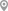Btm Layout 2nd Stage, BangaloreBtm Layout 2nd Stage, BangaloreBtm Layout 2nd Stage, BangaloreBtm Layout 2nd Stage, BangaloreBtm Layout 2nd Stage, BangaloreBtm Layout 2nd Stage, Bangalore

X

Post your Learning Need

Let us shortlist and give the best tutors and institutes.

or

Send Enquiry to Swapna S.

Let Swapna S. know you are interested in their class

Reply to 's review